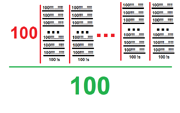11,055 PagesVisual representation of Tetfactor

The Tetfactor is equal to 100!4 using Extensible ! Notation. The term was coined by Yabuszko

## Etymology

The name of this number is based on Greek prefix "tetra-" and the factor.

## Approximations

Notation Approximation
Arrow Notation $$82\uparrow\uparrow\uparrow\uparrow101$$
Chained Arrow Notation $$82 \rightarrow 101 \rightarrow 4$$
BEAF $$\{82,101,4\}$$
Hyper-E notation

$$E160\#100\#100\#100$$

Hyperfactorial Array Notation $$100!3$$
Strong Array Notation $$s(82,100,4)$$
Nested Factorial Notation $$100!$$
Fast-growing hierarchy $$f_5(100)$$
Hardy hierarchy $$H_{\omega^5}(100)$$
Slow-growing Hierarchy $$g_{\eta_{0}}(100)$$

## Sources

Community content is available under CC-BY-SA unless otherwise noted.